Home > A2C > Chapter 8 > Lesson 8.1.6 > Problem8-95

8-95.
1. Evaluate each expression without using a calculator or changing the form of the expression. Homework Help ✎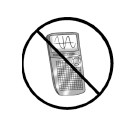1. log(10)

2.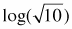3. log(0)

4. 10(2/3)log(27)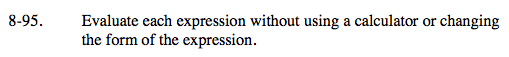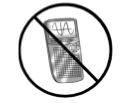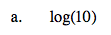Remember that log(x) is the same as log10(x).

1

$\text{log\sqrt{10}=log10^{\frac{1}{2}}}$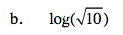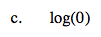10x = 0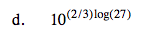Rewrite the exponent using the Product Property of Logarithms. Then simplify using fractional exponents.

Remember that blogb(a) = a.

9